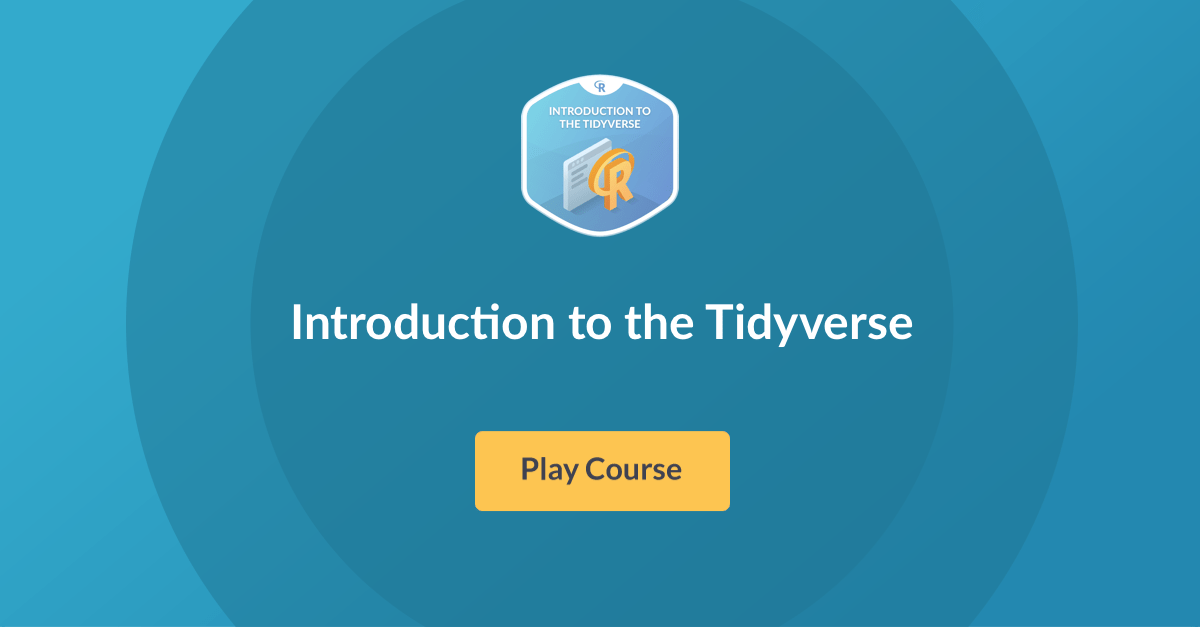Want to share your content on R-bloggers? click here if you have a blog, or here if you don't.

Hi! Big announcement today as we just launched Introduction to the Tidyverse R course by David Robinson!

This is an introduction to the programming language R, focused on a powerful set of tools known as the “tidyverse”. In the course you’ll learn the intertwined processes of data manipulation and visualization through the tools `dplyr` and `ggplot2`. You’ll learn to manipulate data by filtering, sorting and summarizing a real dataset of historical country data in order to answer exploratory questions. You’ll then learn to turn this processed data into informative line plots, bar plots, histograms, and more with the `ggplot2` package. This gives a taste both of the value of exploratory data analysis and the power of tidyverse tools. This is a suitable introduction for people who have no previous experience in R and are interested in learning to perform data analysis.

Take me to chapter 1!

Introduction to the Tidyverse features interactive exercises that combine high-quality video, in-browser coding, and gamification for an engaging learning experience that will make you a Tidyverse expert!What you’ll learn

1. Data wrangling

In this chapter, you’ll learn to do three things with a table: filter for particular observations, arrange the observations in a desired order, and mutate to add or change a column. You’ll see how each of these steps lets you answer questions about your data.

2. Data visualization

You’ve already been able to answer some questions about the data through `dplyr`, but you’ve engaged with them just as a table (such as one showing the life expectancy in the US each year). Often a better way to understand and present such data is as a graph. Here you’ll learn the essential skill of data visualization, using the `ggplot2` package. Visualization and manipulation are often intertwined, so you’ll see how the `dplyr` and `ggplot2` packages work closely together to create informative graphs.

3. Grouping and summarizing

So far you’ve been answering questions about individual country-year pairs, but we may be interested in aggregations of the data, such as the average life expectancy of all countries within each year. Here you’ll learn to use the group by and summarize verbs, which collapse large datasets into manageable summaries.

4. Types of visualizations

You’ve learned to create scatter plots with `ggplot2`. In this chapter you’ll learn to create line plots, bar plots, histograms, and boxplots. You’ll see how each plot needs different kinds of data manipulation to prepare for it, and understand the different roles of each of these plot types in data analysis.

Master the Tidyverse with our course Introduction to the Tidyverse